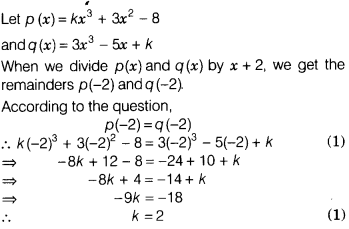# The polynomials kx3 +3x2 -8 and 3x3 -5x + k are divided by x + 2.find the value of k

The polynomials kx3 +3x2 -8 and 3x3 -5x + k are divided by x + 2. If the remainder in each case is the same, then find the value of k.